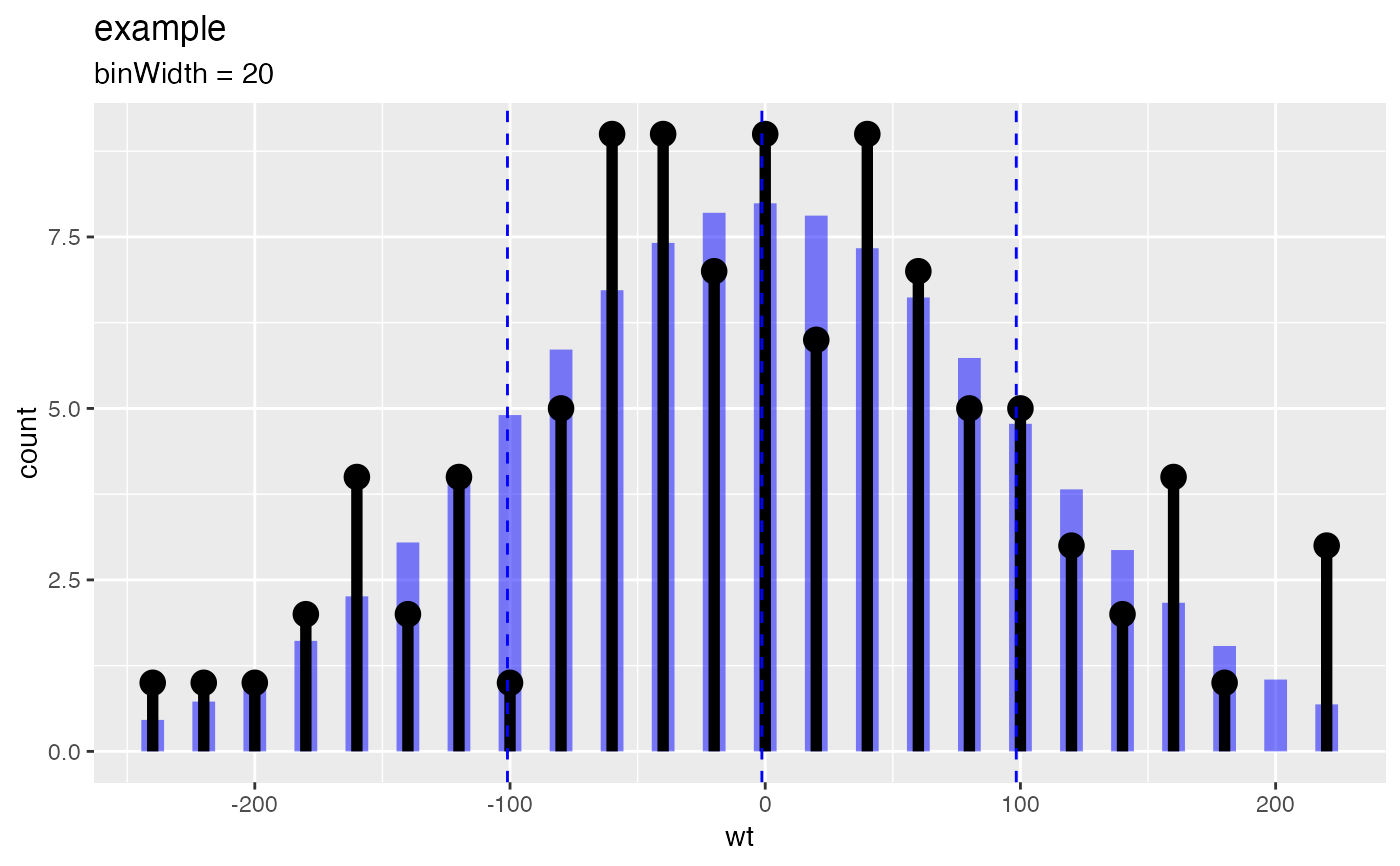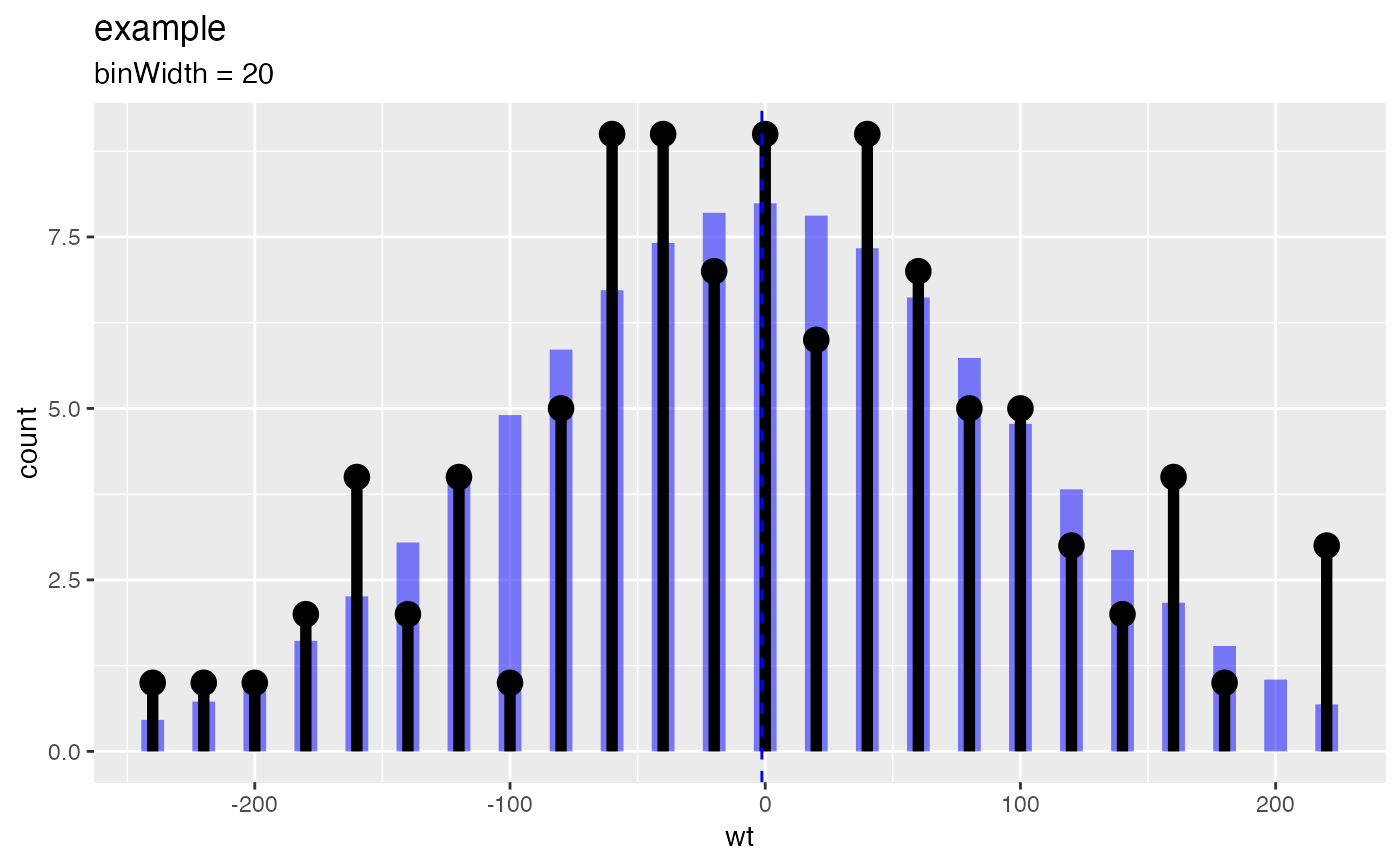Compares empirical data to a normal distribution with the same mean and standard deviation.

PlotDistCountNormal(
frm,
xvar,
title,
...,
binWidth = c(),
hist_color = "black",
normal_color = "blue",
mean_color = "blue",
sd_color = "blue"
)

## Arguments

frm data frame to get values from name of the independent (input or model) column in frame title to place on plot no unnamed argument, added to force named binding of later arguments. width of histogram bins color of empirical histogram color of matching theoretical normal color of mean line color of 1-standard deviation lines (can be NULL)

## Details

Plots the histograms of the empirical distribution and of the matching normal distribution. Also plots the mean and plus/minus one standard deviation.

Bin width for the histogram is calculated automatically to yield approximately 50 bins across the range of the data, unless the binWidth argument is explicitly passed in. binWidth is reported in the subtitle of the plot.

## Examples


set.seed(52523)
d <- data.frame(wt=100*rnorm(100))
PlotDistCountNormal(d,'wt','example')# no sd lines
PlotDistCountNormal(d, 'wt', 'example', sd_color=NULL)